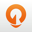### 1. 排序算法基础

#### 1.2 如何分析一个排序算法

1. 最好情况、最坏情况、平均时间复杂度
2. 时间复杂度的系数、常数、低阶
3. 比较次数交换（或移动）次数

#### 1.5 有序度/逆序度

1. 有序度

``````有序元素对：a[i] <= a[j], 如果 i < j
``````

2. 逆序度

``````逆序元素对：a[i] > a[j], 如果 i < j
``````

3. 有序度和逆序度之间的关系

``````逆序度 = 满有序度 - 有序度
``````

### 2. 冒泡排序

#### 2.3 算法分析

1. 冒泡的过程只涉及相邻数据的交换操作，只需要常量级的临时空间，所以它的空间复杂度`O(1)`，是一个原地排序算法
2. 在冒泡排序中，只有交换才可以改变两个元素的前后顺序。为了保证冒泡排序算法的稳定性，当有相邻的两个元素大小相等的时候，我们不做交换，相同大小的数据在排序前后不会改变顺序，因此冒泡排序是稳定的排序算法
3. 冒泡排序的最好情况是要排序的数据已经是有序的了，只需要进行一次冒泡操作，时间复杂度为`O(n)`。而最坏情况是要排序的数据刚好都是倒序的，需要进行`n`次冒泡操作，所以最坏情况时间复杂度为`O(n^2)`

• 最坏情况下，初始状态的有序度为`0`，要进行`n*(n-1)/2`次交换
• 最好情况下，初始状态的有序度为`n*(n-1)/2`不需要进行交换
• 取两者中间值`n*(n-1)/4`，来表示初始有序度既不是很高也不是很低平均情况

#### 2.4 Java 实现

``````// 冒泡排序，a 表示数组，n 表示数组大小
public void bubbleSort(int[] a, int n) {
if (n <= 1) return;

for (int i = 0; i < n; ++i) {
// 提前退出冒泡循环的标志位
boolean flag = false;
for (int j = 0; j < n - i - 1; ++j) {
if (a[j] > a[j+1]) { // 交换
int tmp = a[j];
a[j] = a[j+1];
a[j+1] = tmp;
flag = true;  // 表示有数据交换
}
}
if (!flag) break;  // 没有数据交换，提前退出
}
}
``````

#### 2.5 Go 实现

``````// 冒泡排序，a 表示数组，n 表示数组大小
func BubbleSort(a []int, n int) {
if n <= 1 {
return
}
for i := 0; i < n; i++ {
// 提前退出标志
flag := false
for j := 0; j < n-i-1; j++ {
if a[j] > a[j+1] {
a[j], a[j+1] = a[j+1], a[j]
//此次冒泡有数据交换
flag = true
}
}
// 如果没有交换数据，提前退出
if !flag {
break
}
}
}
``````

### 3. 插入排序

#### 3.1 基本思路

• 对于不同的查找插入点方法（从头到尾、从尾到头），元素的比较次数是有区别的
• 对于一个给定的初始序列移动操作的次数总是固定的，就等于逆序度

#### 3.2 算法分析

1. 插入排序算法的运行并不需要额外的存储空间空间复杂度`O(1)`，也是一个原地排序算法
2. 对于值相同的元素，我们可以选择将后面出现的元素，插入到前面出现元素的后面，这样就可以保持原有的前后顺序不变，所以插入排序也是稳定的排序算法
3. 最好情况下，数据全部有序，只需要从尾到头遍历所有数据，时间复杂度为`O(n)`最坏情况下，数据全部逆序，时间复杂度为`O(n^2)`。总的来看，平均时间复杂度`O(n^2)`

#### 3.3 Java 实现

``````// 插入排序，a 表示数组，n 表示数组大小
public void insertionSort(int[] a, int n) {
if (n <= 1) return;

for (int i = 1; i < n; ++i) {
int value = a[i];
int j = i - 1;
// 查找插入的位置
for (; j >= 0; --j) {
if (a[j] > value) {
a[j+1] = a[j];  // 数据移动
} else {
break;
}
}
a[j+1] = value; // 插入数据
}
}
``````

#### 3.4 Go 实现

``````// 插入排序，a 表示数组，n 表示数组大小
func InsertionSort(a []int, n int) {
if n <= 1 {
return
}
for i := 1; i < n; i++ {
value := a[i]
j := i - 1
// 查找要插入的位置并移动数据
for ; j >= 0; j-- {
if a[j] > value {
a[j+1] = a[j]
} else {
break
}
}
a[j+1] = value
}
}
``````

### 4. 选择排序

#### 4.2 算法分析

1. 选择排序空间复杂度`O(1)`，也是一种原地排序算法
2. 最好、最坏、平均时间复杂度都为`O(n^2)`
3. 选择排序是一种不稳定的排序算法，原因在于每次都要找剩余未排序元素中的最小值，并和前面的元素交换位置，这样破坏了稳定性

#### 4.3 Java 实现

``````// 选择排序，a 表示数组，n 表示数组大小
public static void selectionSort(int[] a, int n) {
if (n <= 1) return;

for (int i = 0; i < n - 1; ++i) {
// 查找最小值
int minIndex = i;
for (int j = i + 1; j < n; ++j) {
if (a[j] < a[minIndex]) {
minIndex = j;
}
}
// 交换
if (minIndex != i) {
int tmp = a[i];
a[i] = a[minIndex];
a[minIndex] = tmp;
}
}
}
``````

#### 4.4 Go 实现

``````// 选择排序，a 表示数组，n 表示数组大小
func SelectionSort(a []int, n int) {
if n <= 1 {
return
}
for i := 0; i < n; i++ {
// 查找最小值
minIndex := i
for j := i + 1; j < n; j++ {
if a[j] < a[minIndex] {
minIndex = j
}
}
// 交换
if minIndex != i {
a[i], a[minIndex] = a[minIndex], a[i]
}
}
}
``````

### 5. 为何插入比冒泡更受欢迎

• 冒泡排序插入排序不管怎样优化，其元素交换次数是一个固定值，即原始数据的逆序度
• 代码实现上来看，冒泡排序的数据交换比插入排序的数据移动要更复杂交换需要`3`个赋值操作，而移动只需要`1`
``````// 冒泡排序中数据的交换操作：
if (a[j] > a[j+1]) { // 交换
int tmp = a[j];
a[j] = a[j+1];
a[j+1] = tmp;
flag = true;
}

// 插入排序中数据的移动操作：
if (a[j] > value) {
a[j+1] = a[j];  // 数据移动
} else {
break;
}
``````

### 参考文章

1.数据结构与算法之美 | 极客时间2.十大经典排序算法动画与解析，看我就够了！（配代码完全版）| 五分钟学算法